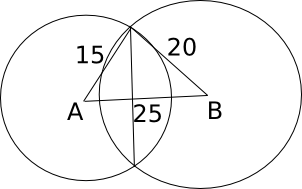Question 10

# The length of the common chord of two circles of radii 15 cm and 20 cm, whose centres are 25 cm apart, is

SolutionThe radii of both the circles and the line joining the centers of the two circles form a right angled triangle. So, the length of the common chord is twice the length of the altitude dropped from the vertex to the hypotenuse.

Let the altitude be h and let it divide the hypotenuse in two parts of length x and 25-x
So, $$h^2 + x^2 = 15^2$$ and $$h^2 + (25-x)^2 = 20^2$$
=> $$225 - x^2 = 400 - x^2 + 50x - 625$$
=> 50x = 450 => x = 9 and h = 12
So, the length of the common chord is 24 cm.